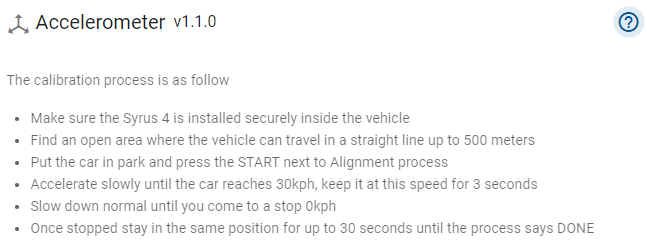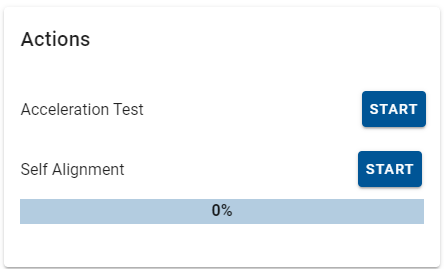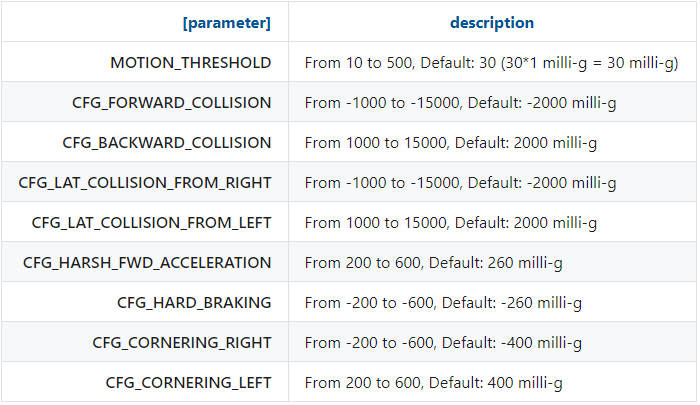• Author
Posts
• #11325
Eugenio Balam
Keymaster

For certain events it is very important to have the Syrus 4 accelerometer properly configured. For example, for aggressive driving events.

Unlike the Syrus 3, the Syrus 4 can be in any position. However, it is required to do a self alignment.

You can rely on the Syrus 4 administration tool. Go to the “Accelerometer” section and press the help button.Now, if you are ready to run the calibration, press the START button.

IMPORTANT: The vehicle must go in a straight line throughout the test.Once the self-alignment is done, we can proceed to configure the thresholds. The following table shows the limits of each parameter.Considerations for choosing the appropriate thresholds:

• Values close to zero indicate higher sensitivity, further ones, lower sensitivity.
• You can get this path to choose a value to start.

We can apply a little math to get an approximate value. Suppose we consider a harsh acceleration when a vehicle goes from 0 kph to 40 kph in 3.95 secs. First, convert speed units in m/s (Divide Kph by 3.6) → 11.11 m/s then, divide it by the seconds → 2.812 m/s^2 last, divide it by g force (9.81 m/s^2) and multiply the result by 1000 → 286 mili-g

You can use the same logic for harsh braking.

Harsh cornering left is not easy to calculate because you need an estimated radius and velocity. For example, suppose we consider harsh cornering driving at 32 kph in a curve with 17m of radius. First, convert kph to m/s → 8.88 m/s, square it and divide it by radius → 4.64 m/s^2, finally divide it by g force and multiply it by 1000 → 473 mili g.

You can take this same value but in the negative for harsh cornering right.

These are some examples of configurations successfully tested on a Chevrolet Suburvan SUV vehicle:

set accelerometer CFG_HARD_BRAKING -300
set accelerometer CFG_HARSH_FWD_ACCELERATION 240
set accelerometer CFG_CORNERING_RIGHT -300
set accelerometer CFG_CORNERING_LEFT 300

For more details on the accelerometer see the manuals:

Syrus 4 Accelerometer